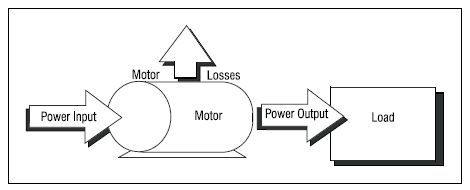# How To Determine The Efficiency of An Electric Motor Using Prony Brake

## Introduction

Efficiency of motors are variable base on the percentage of loads that the motor carried in in a certain period of time. Typically AC motors are designed to operate between 50% to 100 % of the rated load. However the maximum efficiency of an electric motor can be attained when it is operating at 75% and beyond that the efficiency of the motor start to decrease. For example a 10 HP motor can attain the peak efficiency when the load is 7.5 HP.Figure 1. Sample Efficiency Graph of Electric Motors

## How to calculate motor efficiency?

By definition motor is an electrical machine that converts electrical energy to mechanical energy. Therefore we can say that in finding its efficiency we need to know how much electrical input is being used for a given mechanical power it produced.Figure 2. Depiction of motor operation (US Dept. of Energy)

## Prony Brake Test

Prony brake is an equipment that can be use to measure the torque in the shaft of the motor.
• Using set of voltmeter, ammeter and PF meter, connect the leads of the motor to the proper terminals of this meter. Or if there is an available wattmeter, use this equipment measure the power consumption of the motor.
• Connect the prony brake to the shaft of the motor.Figure 3. Prony Brake (Hampden Training Equipment)

• Start the motor without load (meaning the prony brake load mechanism induce zero  torque during this time) and let the motor run to its rated speed,
• Slowly while the motor is running at full speed activate the load mechanism adjust of the prony brake to induce load to the motor (either belt system, magnetic type or hydraulic type).

### To determine the efficiency of the motor at any load (below its rated load) do the following steps:

1. Adjust the load mechanism of the prony brake to attain the percentage load of the motor.

Use the formula:
• HP = Torque x RPM/ 5252
• Where: Power = Horsepower; Torque = ft-lb; Speed = RPM
Example:

Determine the efficiency of the 20 HP, 4 pole motor when it is running at 25% of its rated load.
Note: when the motor is loaded with 25% of its rated load the RPM drops to 1700
• % Load = 20 HP x 25% = 5 HP
• Therefore the torque needed is, T = (HP x 5252) / RPM
• T = (5 HP x 5252) / 1700 = 15.44 ft-lb
• note: use tachometer to determine the actual speed while the motor is loaded w/ 25% of its rated load.
2. After finding the torque at 25% of its rated load, check the wattmeter reading or the voltmeter, ammeter and pf meter reading combination.

Case 1: Using Wattmeter
• Pout = 5 HP
• Convert 5 HP to Watts = 5 x 746 = 3730
• efficiency = 3730 W / Wattmeter reading x 100%
Case 2: Using voltmeter, ammeter and pf meter combination
• Pin = 1.73 x V x I x pf (for three phase motors)
• Pin = V x I x pf (for single phase motors)
• efficiency = 3730 W / Pin x 100%
note: the value of V, I and pf are the actual reading of the meter while the motor is running.

3. Repeat the same process if you want to know the efficiency of the motor say at 30%, 70% or 100%.

## Type of Losses

The losses associated with the operation of electric motors are
• Stator copper loss - due to conductors in the stator
• Rotor copper loss - due to conductors in the rotor
• Core loss - due to magnetic losses in the core of the motor.
• Friction losses - due to the physical contacts when the motor is rotating.
There are several factors why the efficiency of the motor decrease as the time goes by and it cannot attain the original efficiency as designed. For example:
1. Due to aging of the motor sometime the alignment of the rotor is no longer similar with the original thus the friction losses of the motor increase.
2. When the motor was burnt and was subjected for repair then the quality of conductors were not similar to the original.
3. The core of the motor deteriorated.

## Summary

•  Prony brake test is one of the methods to determine the actual efficiency of an electric motor.
• The longevity of the motor and improper repair could decrease the efficiency of the motor.
• Proper preventive maintenance can keep the motor efficiency closely similar similar to the original efficiency.

## References

• Design Assistance Corporation
• Feedback Training Equipment
• US Department of Energy